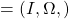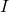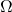# Tykhonov triples, well-posedness and convergence results

### Full PDFcarpathian_2021_37_1_135_143

In this paper we present a unified theory of convergence results in the study of abstract problems.
To this end we introduce a new mathematical object, the so-called Tykhonov triple, constructed by using a set of parameters, a multivalued functionand a set of sequences. Given a problemand a Tykhonov triple, we introduce the notion of well-posedness of problemwith respect toand provide several preliminary results, in the framework of metric spaces.
Then we show how these abstract results can be used to obtain various convergences
in the study of a nonlinear equation in reflexive Banach spaces.

## Additional Information

Author(s) Sofonea, Mircea,  Xiao, Yi-bin